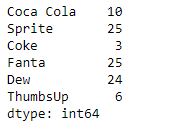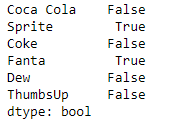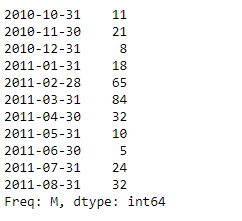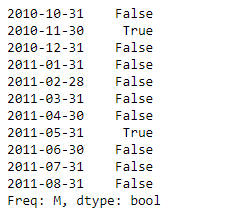# Python | Pandas Series.isin()

• Last Updated : 01 Oct, 2021

Pandas series is a One-dimensional ndarray with axis labels. The labels need not be unique but must be a hashable type. The object supports both integer- and label-based indexing and provides a host of methods for performing operations involving the index.

Pandas Series.isin() function check whether values are contained in Series. It returns a boolean Series showing whether each element in the Series matches an element in the passed sequence of values exactly.

Attention geek! Strengthen your foundations with the Python Programming Foundation Course and learn the basics.

To begin with, your interview preparations Enhance your Data Structures concepts with the Python DS Course. And to begin with your Machine Learning Journey, join the Machine Learning - Basic Level Course

Syntax: Series.isin(values)
Parameter :
values : The sequence of values to test.
Returns : isin : Series (bool dtype)

Example #1: Use Series.isin() function to check if the passed values in the list are contained in the series object.

## Python3

 `# importing pandas as pd``import` `pandas as pd` `# Creating the Series``sr ``=` `pd.Series([``10``, ``25``, ``3``, ``25``, ``24``, ``6``])` `# Create the Index``index_ ``=` `[``'Coca Cola'``, ``'Sprite'``, ``'Coke'``, ``'Fanta'``, ``'Dew'``, ``'ThumbsUp'``]` `# set the index``sr.index ``=` `index_` `# Print the series``print``(sr)`

Output :Now we will use Series.isin() function to check whether the passed values are contained in the series object.

## Python3

 `# check if 25 is present``# in the series``result ``=` `sr.isin([``25``])` `# Print the result``print``(result)`

Output :As we can see in the output, the Series.isin() function has returned an object containing boolean values. All values have been mapped to True if it is present in the list else False.

Example #2 : Use Series.isin() function to check if the passed values in the list are contained in the series object.

## Python3

 `# importing pandas as pd``import` `pandas as pd` `# Creating the Series``sr ``=` `pd.Series([``11``, ``21``, ``8``, ``18``, ``65``, ``84``, ``32``, ``10``, ``5``, ``24``, ``32``])` `# Create the Index``index_ ``=` `pd.date_range(``'2010-10-09'``, periods ``=` `11``, freq ``=``'M'``)` `# set the index``sr.index ``=` `index_` `# Print the series``print``(sr)`

Output :Now we will use Series.isin() function to check whether the passed values are contained in the series object.

## Python3

 `# check if 21 and 10 is present``# in the series``result ``=` `sr.isin([``21``, ``10``])` `# Print the result``print``(result)`

Output :As we can see in the output, the Series.isin() function has returned an object containing boolean values. All values have been mapped to True if it is present in the list else False.

My Personal Notes arrow_drop_up